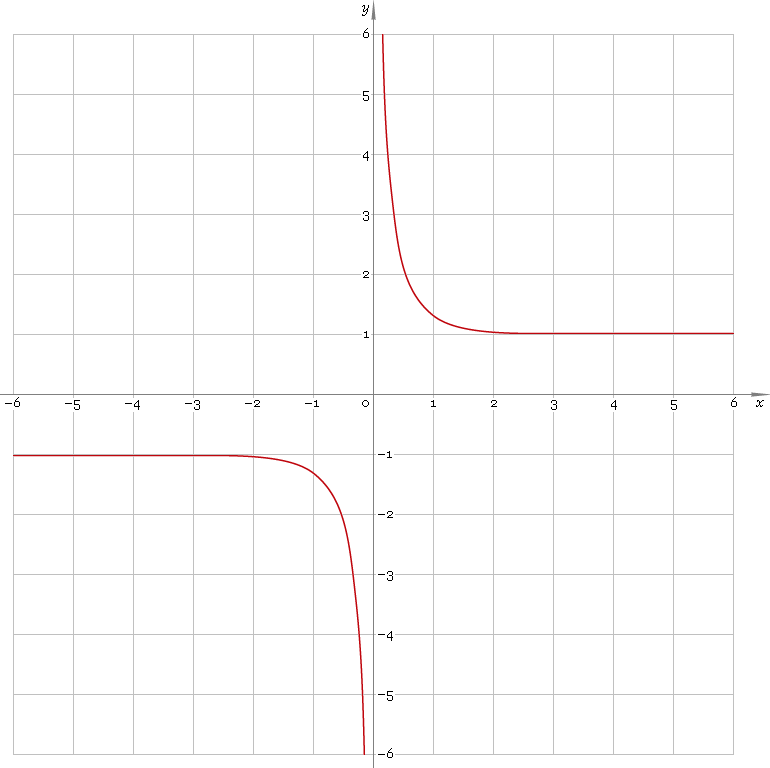The Art of Interface

# coth or cth — hyperbolic cotangent function

Category. Mathematics.

Abstract. Hyperbolic cotangent: definition, plot, properties and identities.

## 1. Definition

Hyperbolic cotangent is defined as

cothx ≡ (ex + ex) /(ex − ex)

## 2. Plot

Hyperbolic cotangent is antisymmetric function defined everywhere on real axis, except its singular point 0 — so, function domain is (−∞, 0)∪(0, +∞). Its plot is depicted below — fig. 1.Fig. 1. Plot of the hyperbolic cotangent function y = cothx.

Function codomain is entire real axis with gap in the middle: (−∞, −1)∪(1, +∞).

## 3. Identities

Base:

coth2x − csch2x = 1

By definition:

cothx ≡ coshx /sinhx ≡ 1 /tanhx

Property of antisymmetry:

coth−x = −cothx

Half-argument:

coth(x/2) = (1 + coshx) /sinhx
coth(x/2) = sinhx /(coshx − 1)
cothx = [1 + tanh2(x/2)] /[2 tanh(x/2)]

Doulbe argument:

coth(2x) = (coth2x + 1) /(2 cothx)

Triple argument:

coth(3x) = (coth3x + 3 cothx) /(3 coth2x + 1)

coth(4x) = (coth4x + 6 coth2x + 1) /(4 coth3x + 4 cothx + 1)

Power reduction:

coth2x = (cosh(2x) + 1) /(cosh(2x) − 1)
coth3x = (cosh(3x) + 3 coshx) /(sinh(3x) − 3 sinhx)
coth4x = (cosh(4x) + 4 cosh(2x) + 3) /(cosh(4x) − 4 cosh(2x) + 3)
coth5x = (cosh(5x) + 5 cosh(3x) + 10 coshx) /(sinh(5x) − 5 sinh(3x) + 10 sinhx)

Sum and difference of arguments:

coth(x + y) = (1 + cothx cothy) /(cothx + cothy)
coth(xy) = (1 − cothx cothy) /(cothx − cothy)

Product:

cothx cothy = [cosh(x + y) + cosh(xy)] /[cosh(x + y) − cosh(xy)]

Sum:

cothx + cothy = sinh(x + y) /(sinhx sinhy)
cothx − tanhy = sinh(yx) /(sinhx sinhy)

## 4. Support

Hyperbolic cotangent function coth or cth of the real argument is supported by free version of the Librow calculator.

Hyperbolic cotangent function coth or cth of the complex argument is supported by professional version of the Librow calculator.

## 5. How to use

To calculate hyperbolic cotangent of the number:

``coth(-1);``

To calculate hyperbolic cotangent of the current result:

``coth(rslt);``

To calculate hyperbolic cotangent of the number x in memory:

``coth(mem[x]);``